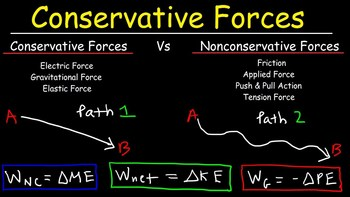# Conservative and Non-conservative ForcesConservative Forces

A force is said to be conservative if work done by the force or against the force on moving a body depends only on initial and final position of the body and is independent on the nature of the path followed between the two positions. For example, gravitational force, elastic force In a stretched or compressed spring, electrostatic force between two stationary charges.

Suppose a body is moved from point A to point B and the again from point B to A. Here, if the force used is conservative then,

W(A to B) = - W(B to A)

∴         W(A to B) + W(B to A) = 0

This shows that the work done by a conservative force on moving a  body in a complete closed path is always zero.

Non – Conservative forces

A force is said to be non-conservative if work done by the force or against the force on moving a body is dependent on the path followed between the initial and final positions. Example: the frictional forces, the velocity dependent forces such as air resistance, viscous force etc are non – conservative forces.

For a non-conservative force F, W(A to B)-W(B to A)

∴         W(A to B) + W(B to A)0

This shows that the work done by a non-conservative force on moving a body in a complete closed path is not zero.